Ex 12.2

Chapter 12 Class 10 Areas related to Circles (Term 1)
Serial order wise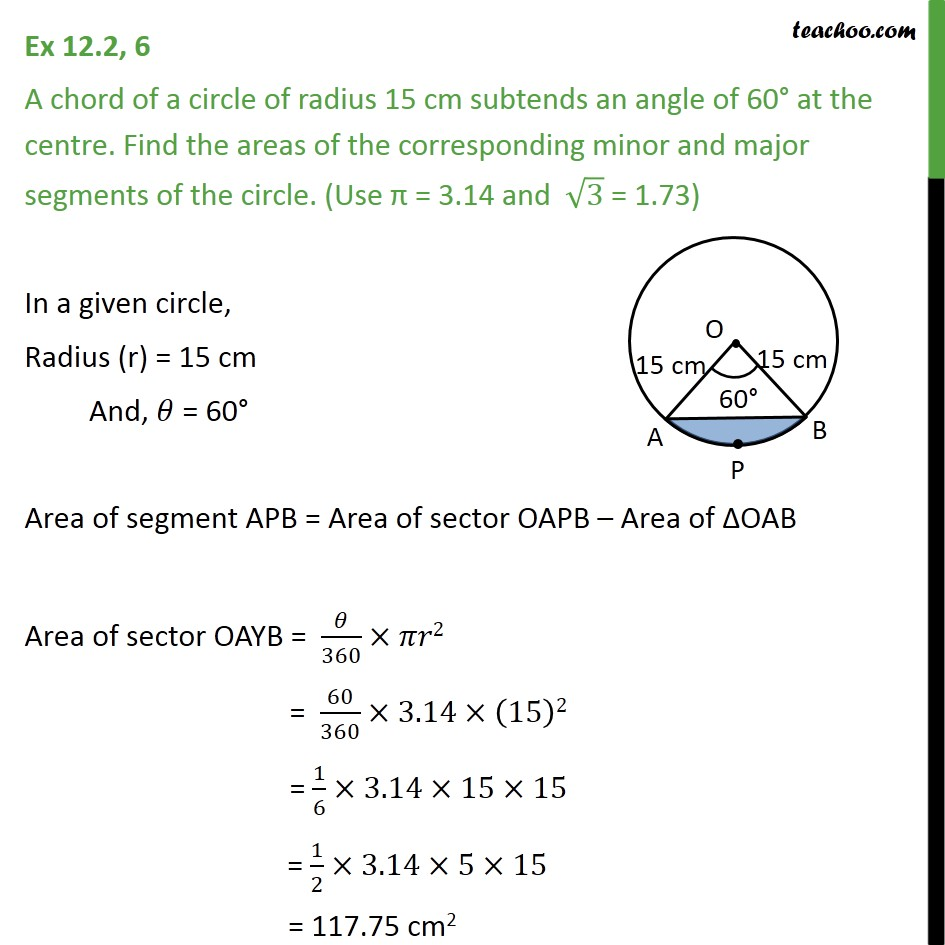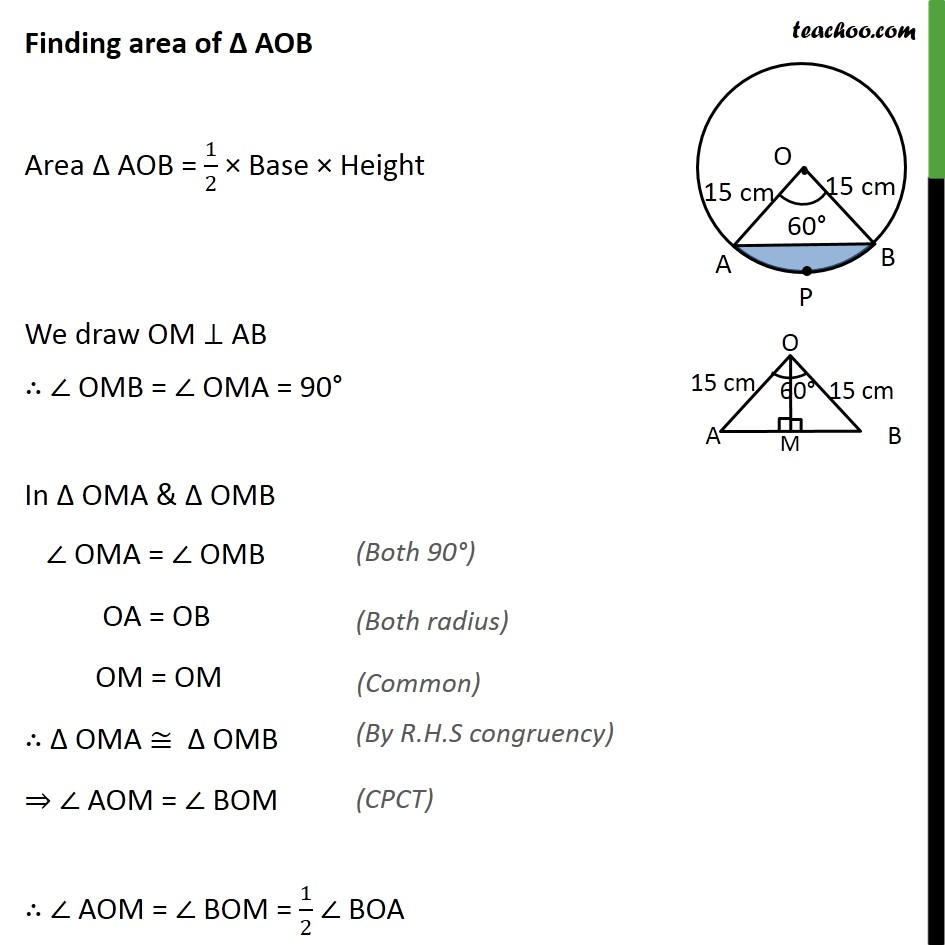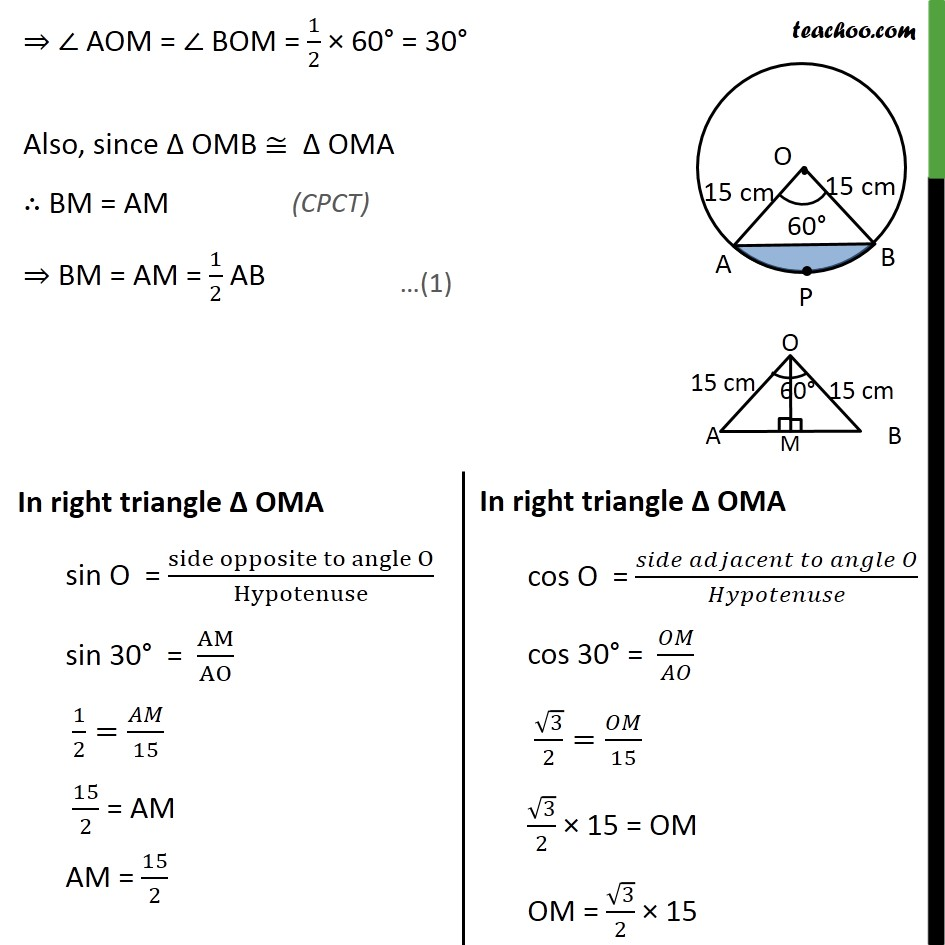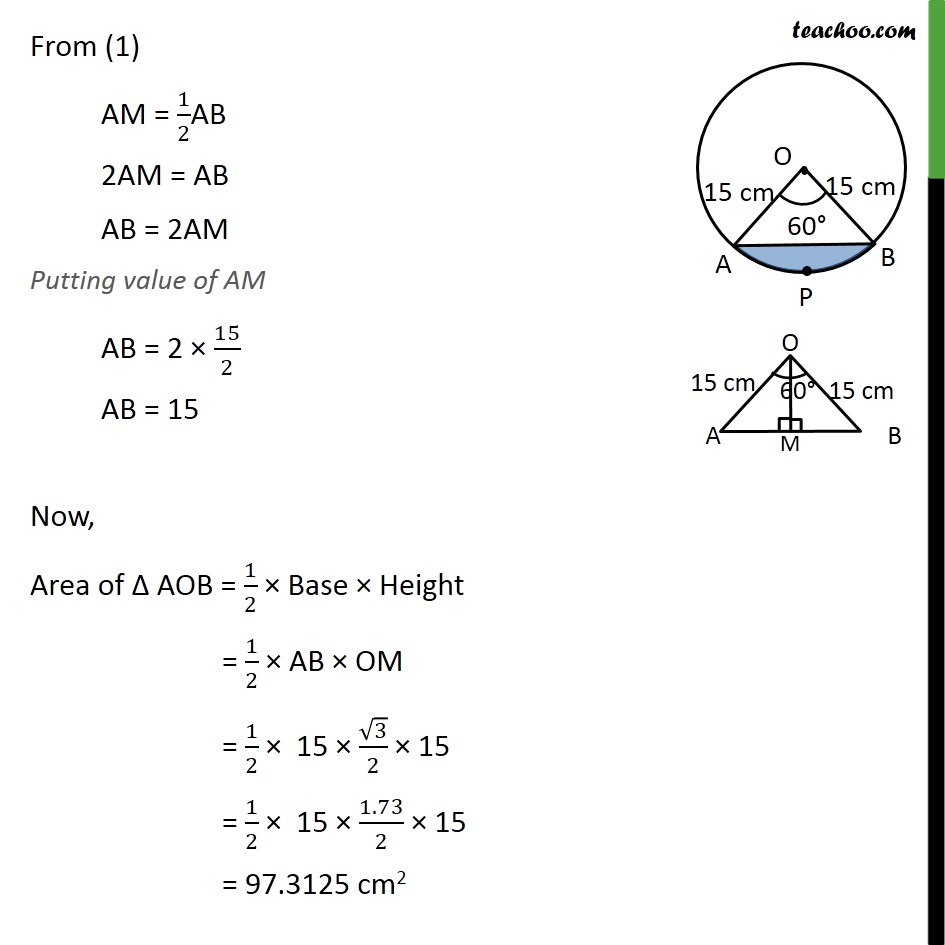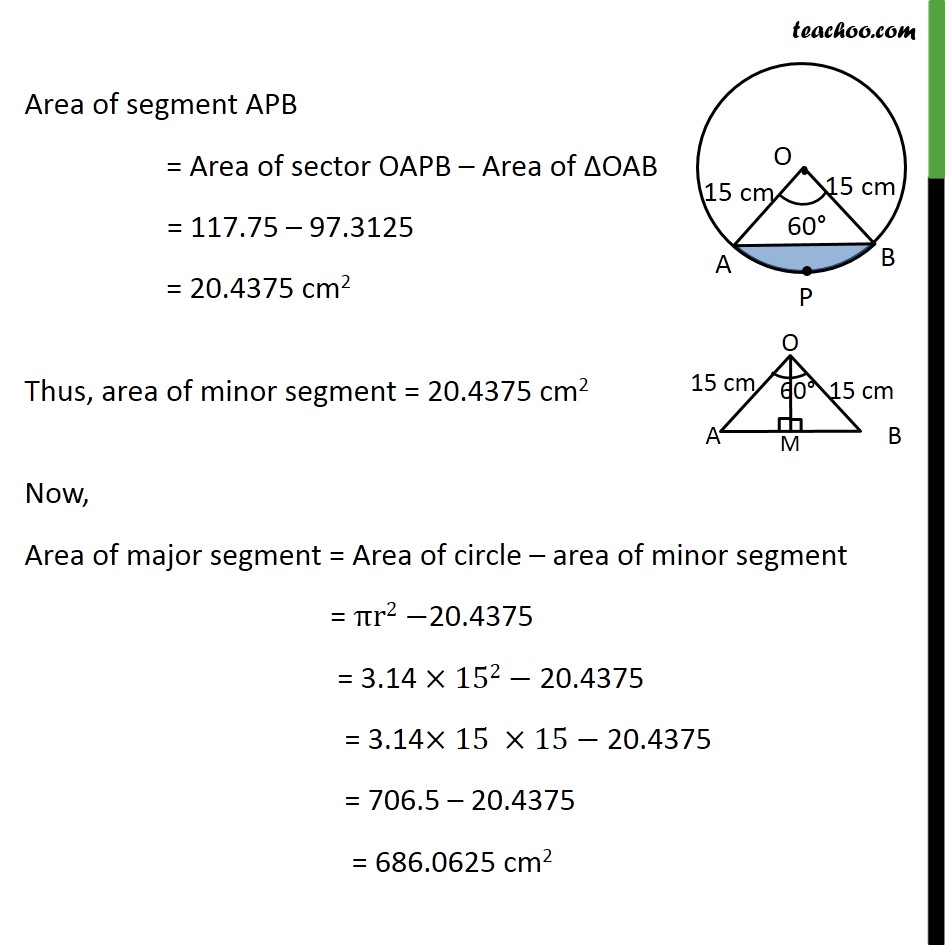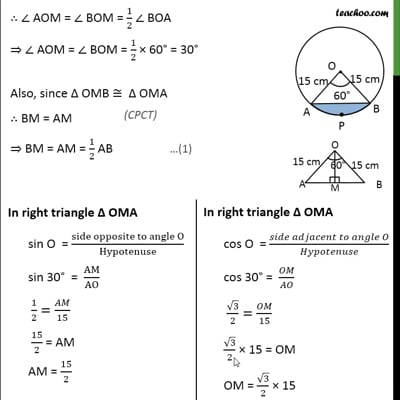This video is only available for Teachoo black users

### Transcript

Ex 12.2, 6 A chord of a circle of radius 15 cm subtends an angle of 60° at the centre. Find the areas of the corresponding minor and major segments of the circle. (Use π = 3.14 and √3 = 1.73) In a given circle, Radius (r) = 15 cm And, 𝜃 = 60° Area of segment APB = Area of sector OAPB – Area of ΔOAB Area of sector OAYB = 𝜃/360×𝜋𝑟2 = 60/360×3.14×(15)2 = 1/6×3.14×15×15 = 1/2×3.14×5×15 = 117.75 cm2 Finding area of Δ AOB Area Δ AOB = 1/2 × Base × Height We draw OM ⊥ AB ∴ ∠ OMB = ∠ OMA = 90° In Δ OMA & Δ OMB ∠ OMA = ∠ OMB OA = OB OM = OM ∴ Δ OMA ≅ Δ OMB ⇒ ∠ AOM = ∠ BOM ∴ ∠ AOM = ∠ BOM = 1/2 ∠ BOA ⇒ ∠ AOM = ∠ BOM = 1/2 × 60° = 30° Also, since Δ OMB ≅ Δ OMA ∴ BM = AM ⇒ BM = AM = 1/2 AB From (1) AM = 1/2AB 2AM = AB AB = 2AM Putting value of AM AB = 2 × 15/2 AB = 15 Now, Area of Δ AOB = 1/2 × Base × Height = 1/2 × AB × OM = 1/2 × 15 × √3/2 × 15 = 1/2 × 15 × 1.73/2 × 15 = 97.3125 cm2 Area of segment APB = Area of sector OAPB – Area of ΔOAB = 117.75 – 97.3125 = 20.4375 cm2 Thus, area of minor segment = 20.4375 cm2 Now, Area of major segment = Area of circle – area of minor segment = πr2−20.4375 = 3.14 ×152− 20.4375 = 3.14×15 ×15−"20.4375" = 706.5 – 20.4375 = 686.0625 cm2